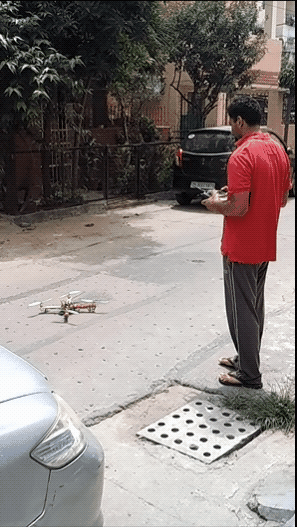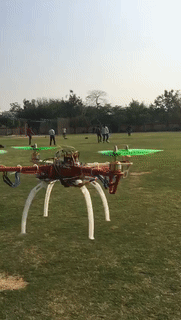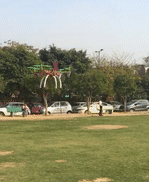top of page
Search
• sidharthtalia

# The journey begins!

Updated: Dec 1, 2021

## Preface

I consider my first “official” project (the basic robots like line followers being unofficial) in the domain of robotics to be my quadcopter project. My mentors initially gave me this “dare” at my first internship with the expectation that I would use a ready-made open source autopilot like pixhawk (running either px4 or APM). Still, I decided to up the ante and create the flight controller myself for the basic task of attitude and altitude control(using a rangefinder).### A project assembled by you is not a project made by you

My objective was to learn. A ready-made autopilot would have allowed me to finish the project faster and given me an overview of the components involved, like PID and state estimation, but the knowledge would be incomplete; I would not learn the intricate details of these components.

The first step was to get the hardware in order, which includes finding the right frame and propulsion system, and figuring out how things would be put together. The overall idea was to make sure that the quadcopter’s thrust to weight ratio was close to 2:1 and that the wiring was as neat as possible to prevent it from getting caught in the propellers. Note that this wasn’t the first project in which I was using attitude estimation and PID; I had made a 2-wheel balance bot before this and was thus already familiar with the mathematics involved.### The software

After setting up the hardware, I started working on the code. It is essential to set a target refresh rate for your controller. A low refresh rate can cause control as well as estimation issues. The general consensus at the time when I made this project was that an update rate of 200-250 Hz would be required for good control, 250 Hz being the update rate used by the APM running on the Atmega2560. To challenge myself, I set the target refresh rate to 400 Hz, which was the refresh rate available on the more expensive pixhawk 2.4.6 flight controllers. Thus, the time for execution would be 2500 microseconds. However, I couldn’t use all of this time for state estimation and control; some of this time would be used for reading sensors, some for reading manual/user inputs, some for checking failsafe conditions, and most of it for writing PWM outputs to the motors (since I was using a bit-banging based approach). Thus, I was left with a mere 1000 microseconds for state estimation and control.

The structure of the flight control code was as follows:

1. Read sensor data on the I2C (takes ~300 microseconds).

2. Raise all the PWM pin voltages to 5 V with bit-banging (the 1000 microsecond for state estimation and control begins).

3. Preprocess sensor data and estimate yaw, pitch, roll.

4. Calculate controller (PID) outputs for yaw, pitch, and roll (the 1000 microsecond slot ends)

5. Send out control commands (PWM) to electronic speed control units for each motor (using bit-banging).

### State estimation

While I had created a balance bot project before this, the speed of execution wasn't really a concern in that project as the control rate was a mere 50 Hz. The microcontroller being used here was an Atmega328P, which is an 8-bit microcontroller with a clock speed of 16 MHz. For the uninitiated, this is roughly a thousand times slower than your mobile phone’s processor, not in terms of clock speed, but the overall time taken to execute operations. State estimation is the most computationally expensive task of the bunch, as it requires sensor data pre-processing (bias removal and scaling) and trigonometry for measuring the tilt angles.After some testing, I found out that the trigonometric function arcsin(x) was taking a fair amount of computing time; calling the function twice would cost roughly 30% of the time I had for the whole code. In a bid to optimize this, I came up with a simpler formula that sacrificed accuracy in favor of speed:The formula here was simply:

`arcsin(x) = x*(0.94+(0.54*x*x))`

I modeled arcsine(x) as a cubic polynomial to reduce the computation time. This approach was 5 times faster than the average case for arcsin(x). The difference in the angles was only significant after 40 degrees and I did not plan to make the drone tilt itself any more than that, making this sufficiently accurate.

The sensor fusion between the accelerometer and the gyroscope used a modified complementary filter which reduced the weightage given to the accelerometer derived measurements in proportion to the difference between total acceleration and gravitational acceleration. Simply put, the accelerometer derived readings can not be trusted during large accelerations.

### Control System Tuning:

Once the state estimation was out of the way, I started tuning the PID gains. This isn’t as hard on ready-made autopilots as they provide some baseline gains. However, I had to find the baseline myself, and tuning a system with four spinning blades can be... eventful.reference

Joke(r)s aside, the process is pretty simple (inspired by the Ziegler-Nichols method):

1. Set all gains to 0 for roll and pitch (yaw is a first-order system so any small gain should work just fine. Set it at 0 to begin with in case you're not sure what this "small gain" could be).

2. Increase roll and pitch P, D gains by small amounts until it feels like you have good control over the pitch and roll angle. Keep the D gain equal to half the P gain or less. The increment amount can be obtained as: increment = 0.1 * full_scale_output / maximum_expected_error If you're using standard PWM based ESCs, this value is equal to 0.8. Some tutorials say to increase P-gain only at first until the system is "critically stable" (constant amplitude oscillations). However, the quadcopter is a machine with four spinning knives in the air; increasing the gains until the system is “critically stable" does not sound like a good idea for anyone.

3. Reduce the P-gain by 5-6% if it seems to oscillate. Then increase the I gain incrementally until the system shows small, slow oscillations. At this point, reduce the I gain by 50%.

Here's a short gif of the first flight:### I hate compromises

After getting used to flying the quadcopter, I felt that tuning this system was a compromise between speed and steady-state oscillations; a system with high P-gain would be snappy (which I liked) but at the same time nervous (oscillatory) around the steady-state setpoint. A system with a low P-gain would be stable/ have smaller oscillations, but be sluggish in transitions. I did not like this compromise and thus decided to get rid of it.

What I needed was for the gains to adapt in accordance with the kind of dynamics I demand from the system. If I demand large movements, I would like it to increase the gains temporarily, while for smaller movements, I would prefer the smaller gains.

Thus the “Exponential” Integral Derivative controller was created. The way this works is that the P-gain starts from a base value and increases with the square of the error to 1.5 times its base value when the error is 40 degrees (full deflection to one side). One might wonder if this would make the system unstable (and rightly so).The way to tune this system is to first find the baseline PID gains for snappy behavior (as snappy as you can get without large overshoots) and then cut the P-gain by 33%

` Base_P_gain = 0.67*P_gain_snappy`

The P gain is increased according to the following formula:

```K = 0.5/(maximum_error^2)
P_gain = Base_P_gain*(1 + K*(error^2))```

On a full deflection, the P gain would be increased to 1.5 times of its base value, which is:

```P_gain = Base_P_gain*1.5
therefore P_gain = 1.5*0.67*P_gain_snappy = P_gain_snappy```

The P-gain’s base value actually results in an over-damped system as The P-gain is at 70% of the P-gain required for a critically damped system. However, as the error gets close to the maximum_error limit (40 degrees of tilt in this case), the P-gain is increased by 50%, making the system critically damped. The purpose of the exponential component is to provide an overdamped system for small movements and a critically or even underdamped system for large movements.This allows the quadcopter to fly stable like this--And also have a snappy response like this-### And that's a wrap!

The code for the flight controller is available here(although I would advise others to consider it as an experimental project with no guarantees of safety whatsoever). From selecting the right hardware to optimizing the code and adding failsafe measures, the field of robotics expects you to learn it all, which is exactly why I find this field so interesting; being a field made by a combination of different fields, it allows you to learn multiple things as well as understand connections between them.